# An Overview Of Math Symbols & Greek Letters

in StemSocial2 years ago

Hi there. In this post, I would like to cover math symbols and Greek letters.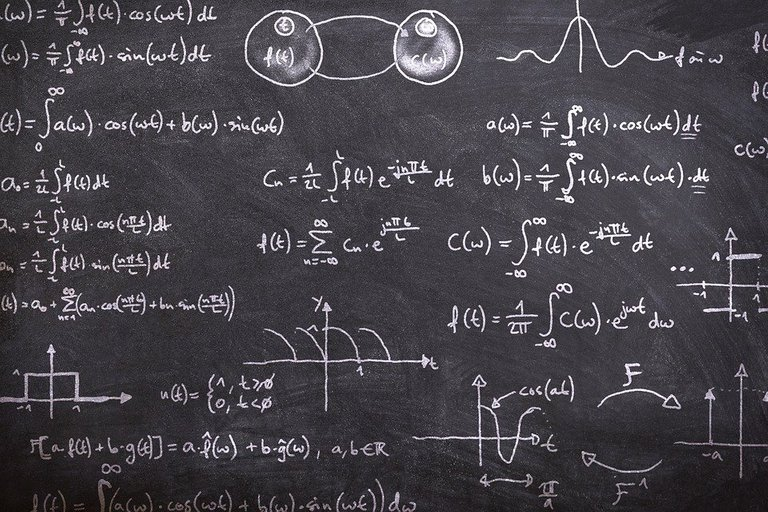Pixabay Image Source

## Topics

• Math Symbols & Greek Letters Not Taught Sometimes
• Basic Math Symbols
• Math Symbols Associated With Numbers
• Other Math Symbols
• Greek Letters & Their Usages
• Notes

## Math Symbols & Greek Letters Not Taught Sometimes

My first encounter with math symbols and Greek letters was from grade 10 mathematics with trigonometry. Outside of pi (π), I think the first Greek letter that I saw in the mathematics space was theta (θ). Theta (θ) is commonly used to symbolize an unknown angle for a right angled triangle with sine, cosine and tangent ratios.

In my first year university math courses, I got confused by my calculus teacher in Calculus I. He was one of those professors who was smart but not great at teaching. He often liked to many math symbols and not explain what they were. It was like as if he expected you to know them right off the bat. Over the years I got used to math notation, math symbols and Greek letters in the math language. The math course on Introduction to Proofs was helpful with understanding some of the symbols (∃, ∀, ∪, ∩, ε, ⊂, ⊆, ⊄, ⊈) at the time.

Looking back, I did wish that there was time being spent in learning the math symbols, Greek letters. If not that, some recommended resources on learning these symbols for mathematics.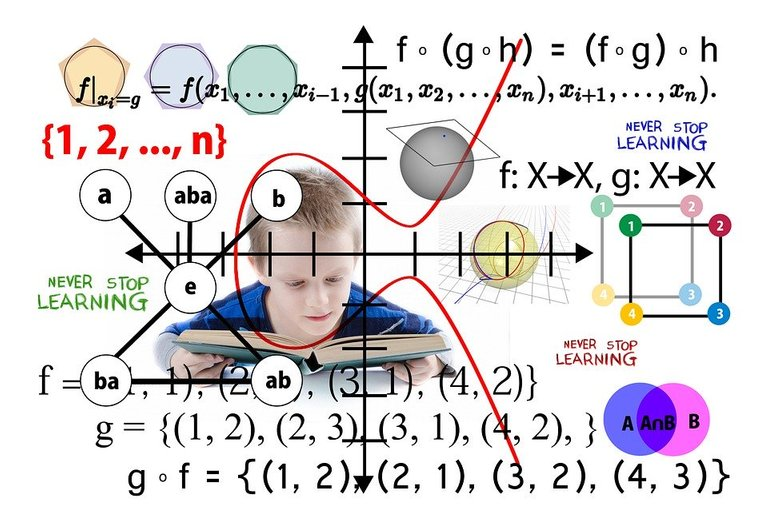Pixabay Image Source

## Basic Math Symbols

Basic math symbols would be the common math symbols that you would see from grade school until high school. We have the basic arithmetic symbols such as `+, -, x and ÷` for addition, subtraction, multiplication and division.

Symbols With Angles

There are a couple of math symbols that are associated with angles such as `∠` and `°`. The little circle represents degrees as in 80 degrees or 80°. This
`∠` symbol represent an angle from a 2d shape. If you have a triangle like ΔABC, the angle A can be represented as `∠A`.

Inequality Signs

The equal sign (=) is well known for many. There are times when you have inequality signs such as `<, >, ≤, ≥` for less than, greater than, less than or equal to or greater than or equal to. As one example `7 > 0` means that 7 is greater than zero. Having `x ≤ -1` means that the variable `x` is less than or equal to -1.

Percent Sign

The percent sign `%` represents a part of the whole. One hundred percent of something represents all of the thing. Anything above 100% represents all of the whole and more. Examples of percents include 25% meaning one quarter of the whole, 50% means half of the whole, 200% means twice of the whole, 10000% means 100 times of the whole.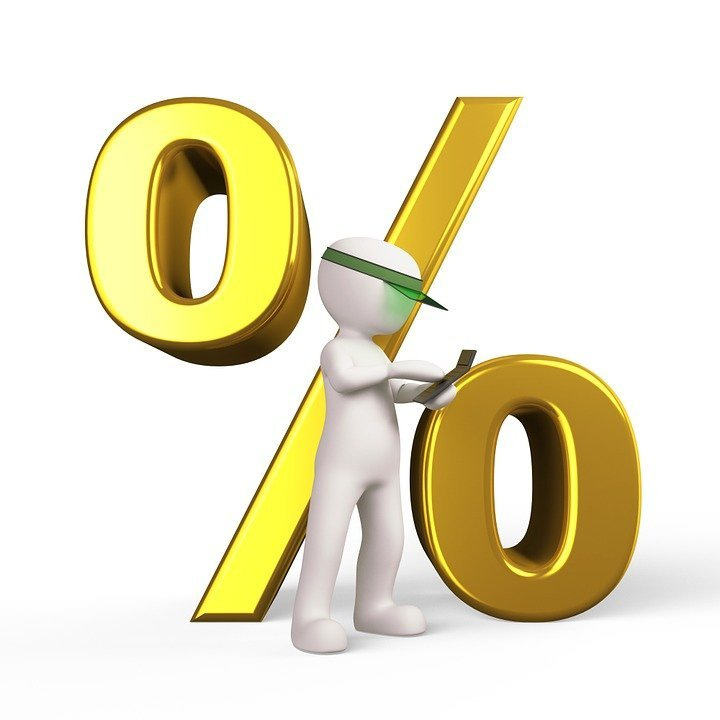Pixabay Image Source

## Math Symbols Associated With Numbers

Numbers are quite popular and well-known in mathematics. At the higher levels of mathematics, there are less numbers and more symbols.

Number Systems

With classifying numbers, we have whole numbers, integers, rational numbers, real numbers and the complex numbers.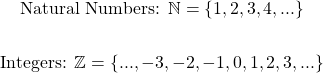Rational numbers include half, three-quarters.

Irrational numbers include π (3.14... ) and square root of 2. Irrational numbers contain an endless amount of digits.

Infinity Symbol

Infinity refers to more of a concept than a number. It refers to something that is endless. The symbol for infinity is `∞`. You can also have `+∞` or `-∞`. Note that I won't go into the concepts of countable and uncountable topics from set theory for this post.

One common usage with infinity is with limits. I list two examples below.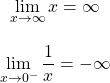Interval Notation

Interval notation is a compact way of expressing inequalities. This is used for domain and range of mathematical functions.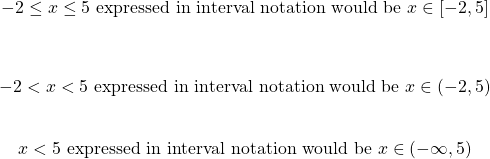Factorials

A factorial is a compact way of express a product of numbers. When I mention product of numbers, I refer to numbers being multiplied together. Given a whole number positive integer `n`, n factorial contains the exclamation mark symbol. The factorial definition is: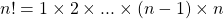For `0!`, it is equal to 1 by definition.Pixabay Image Source

## Other Math Symbols

Sum Sigma Notation

The sum sigma notation is a compact way of expressing the addition of many numbers. I showcase some examples here.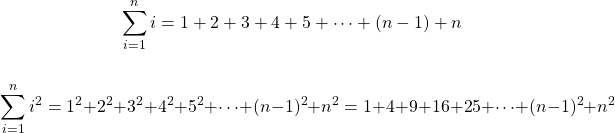Product Pi Notation

You can also express the product of numbers from multiplication with the product pi notation.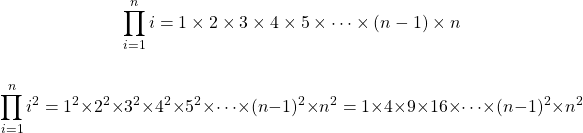Derivatives

From calculus, derivatives are very common. Here are some derivatives examples.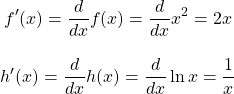From multivariable calculus, we have partial derivatives.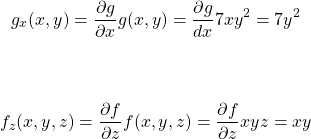Integrals

Integrals from calculus are quite common. They are commonly used to calculate the area under a curve. Here are a few examples.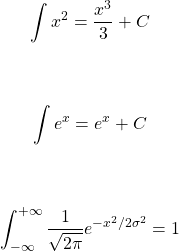This last integral represents the area underneath a standard normal distribution curve being equal to 1.

Logic Symbols

The field of mathematics does contain logic and their symbols. Here are some logic symbols and their meanings.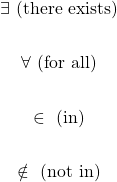Source For this Example: http://mathonline.wikidot.com/the-monotone-convergence-theorem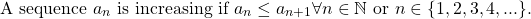Some Set Theory Basics

Set theory is a branch of pure mathematics that focuses on sets, groups and other abstract parts of mathematics. Knowing the basics of set theory helps a bit with logical thinking and for SQL joins.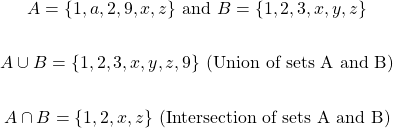Pixabay Image Source

## Greek Letters

I wish someone told back then that a lot of mathematics at the university level and above contained many Greek letters as variables. Greek letters do appear in some economics courses, finance courses, physics courses, college clubs and maybe in some psychology courses that have statistics.

A good webpage resource is https://interestingengineering.com/common-uses-greek-letters-science-maths-engineering. This resource contains Greek letter usage in mathematics and in engineering.

• alpha (α)
• beta (β)
• gamma (γ)
• delta (δ)
• epsilon (ε)
• zeta (ζ)
• eta (η)
• theta (θ)
• lambda (λ)
• pi (π)
• rho (ρ)
• sigma (σ)
• tau (τ)
• phi (φ)
• chi (χ)
• psi (ψ)
• nu (υ)
• omega (ω)
• Gamma (Γ)
• Delta (Δ)
• Omega (Ω)

My background is in mathematics, probability and statistics with some finance. The symbols that I see often are theta (θ), lambda (λ), pi (π), rho (ρ), sigma (σ), tau (τ), phi (φ), chi (χ), Gamma (Γ), Delta (Δ), and omega (ω). Depending on what you study in mathematics, engineering, physics, economics, etc, what you see in Greek letters may vary.

Delta (Δ) is worth a highlight here. The common use case for delta is for a difference of values. A time delta refers to a difference in times. Delta in finance refers to the rate of change between a financial option's price and a \$1 change in the underlying asset. (Reference)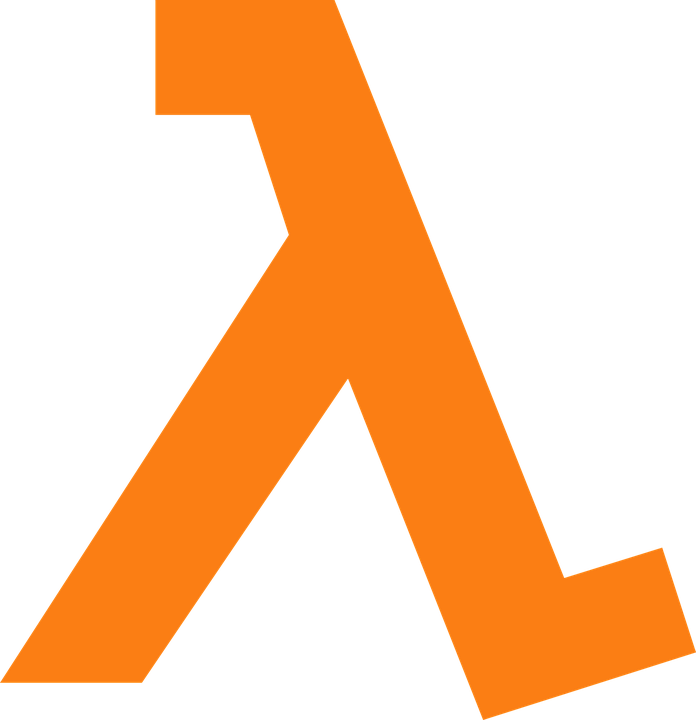Pixabay Image Source

## Notes

The ones displayed here are some of the most common math symbols at the elementary school level, high school level and first year university level. There are many more math symbols out there.

Alot of the math symbols were rendered here with the use of QuickLatex.com. For those not familiar with LaTeX you can use `https://math.typeit.org/`. It is an online keyboard that allows for typing out math symbols.

Sort:
2 years ago

I didn't even know you could type some of these symbols out lol...

I'll definitely keep that site bookmarked.

I copy and paste the math/Greek symbols from the https://math.typeit.org/ website.Thanks for your contribution to the STEMsocial community. Feel free to join us on discord to get to know the rest of us!

Please consider supporting our funding proposal, approving our witness (@stem.witness) or delegating to the @stemsocial account (for some ROI).

Please consider using the STEMsocial app app and including @stemsocial as a beneficiary to get a stronger support.